## Example Questions

### Example Question #1 : How To Factor A Trinomial

Solve each problem and decide which is the best of the choices given.

What are the zeros of the following trinomial?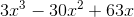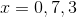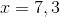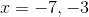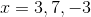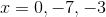First factor out a. Then the factors of the remaining polynomial,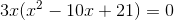, are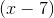and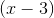.
Set everything equal to zero and you get,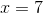, andbecause you cant forget to setequal to zero.